### ICFiles SOC 2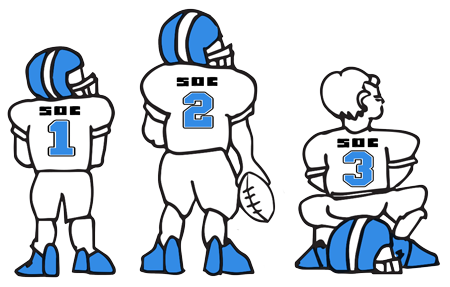#### Secure File Transfer Soc 2 starts at \$1 per month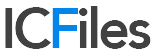# 4 Common Depreciation Methods and Their Uses

August, 2022

## 4 Common Depreciation Methods and Their UsesDepreciation is the accounting concept that evaluates an asset's useful life. As the Internal Revenue Service explains, depreciable property – which could include equipment, structures, means of transportation, fixtures, etc. – is examined to see how many years the purchase price can be averaged and “deducted from taxable income.” This is in contrast to “full expensing,” which allows companies to write off investments straight away. For dual use property (personal and commercial), only the proportion of property that's used for business may be depreciated. Property eligible for depreciation must be owned by the business, be used for business purposes/income-producing activity, and have a determinable useful life.

1. Straight Line Depreciation Method

This method of depreciation determines a constant amount to expense annually over the useful life of the property. It's calculated as follows, with the following example circumstances assumed:

Machinery costing \$50,000 with a life of 12 years and \$2,500 in salvage value.

= (Cost – Salvage Value) / Useful life

= (\$50,000 - \$2,500) / 12

= \$47,500 / 12

= \$3,958.33

Considerations

When implementing this method of depreciation, if the asset's useful life and salvage value is assumed incorrectly, it could skew results. For assets that become outdated prematurely and/or require higher maintenance costs toward the end of its useful life, this method can lead to improper results.

2. Double Declining Balance Depreciation Method

This method, generally speaking, is double that of the straight-line rate.

Annual Depreciation Rate = (100% / Useful life of asset) x 2

Annual Depreciation Rate = (100% / 10) x 2 = 20%

Let's assume that property, plant and equipment (PP&E) costs \$75,000, will produce for 10 years and have a salvage value of \$6,000.

From there, we work to establish the Periodic Depreciation Expense (PDE) = Beginning Book Value x Rate of Depreciation

Using the formula for PDE, we get: \$75,000 x 0.20 (20 percent) = \$ 15,000 for the first year's depreciation expense.

Then, the first year's depreciation expense is subtracted from the item's beginning book value. Ending Book Value = \$75,000 - \$15,000 = \$60,000

To determine each subsequent year's ending book value, it begins with last year's ending book value minus the newly calculated annual depreciation expense.

Year 2 Calculation for Ending Book Value: \$60,000 - (\$60,000 x 0.20 = \$12,000) = \$48,000

Considerations

This method expenses a greater proportion in the earlier years compared to the later years. This is attributable to assets that produce more for a business in their earlier years compared to later years. This method can help businesses depreciate items that lose value quickly, such as electronics, and similar items that become obsolete due to improving technology. It's not necessarily double or 200 percent of the straight-line rate. It could be more or less than double of the straight-line rate. However, the double depreciation rate does remain constant over the depreciation process.

3. Units of Production Depreciation Method

This takes either the amount of discrete time utilized for production or the tally of items to be manufactured with the production equipment subject to depreciation. It's calculated as follows:

Depreciation Expense = ((Cost - Salvage value) / (Life in Number of Units)) x Number of Units Produced During Accounting Time Frame.

Let’s assume a piece of equipment costs \$100,000, has a projected lifetime production ability of 150 million widgets and will salvage for \$10,000. It's projected to create an output of 25 million widgets within the accounting year.

Depreciation Expense = ((\$100,000 - \$10,000) / (150 million)) x 25 million

= ((\$90,000) / (150 million)) x 25 million

= 0.0006 (unit) x 25 million

= \$15,000

Considerations

This method can help businesses such as manufacturers that produce discrete items that can be counted and expensed per piece. Depreciation starts when the manufacturer begins to make items and stops when the unit has produced all of its life's items within a pre-defined time frame.

4. Sum-of-the-Years Digits Depreciation Method

It's calculated as follows:

Remaining Life (RL) of an asset is divided by the sum of the years digits (SYD) x Depreciation Base. The Depreciation Base = (Cost - Salvage Value)

Assuming there are equipment costs of \$50,000, with a useful life of 12 years and a salvage value of \$3,500. Depreciation Base = \$50,000 - \$3,500 = \$46,500

RL = the remaining life of the asset. When the item starts running, it will have 12 years of a remaining life. One year later, or 12 months after usage began, the asset will have 11 years remaining, and so on. For an item with 12 years of useful life, it will be “the sum of the years” or 1+2+3+4+5+6+7+8+9+10+11+12.

The first year of use or the item's Remaining Life will be 12 / 78 = 0.1538. Then 0.1538 x \$46,500 = \$7,153.85.

Year 2 would be calculated as 11 / 78 = 0.1410. Then 0.1410 X \$46,500 = \$6,557.69.

Considerations

This method is another way to speed up the percentage of depreciation sooner, instead of toward the end of the asset's useful life. The longer the asset is used, the less the asset provides utility to the business. Therefore, it helps businesses take advantage of depreciation sooner. It's a trade-off for items that require more maintenance as time goes on, as the item's value drops inversely.

Conclusion

Depending on the type of business and what it produces or provides as a service, understanding how depreciation works can give an accurate picture of the company's finances and help navigate tax laws efficiently.

These articles are intended to provide general resources for the tax and accounting needs of small businesses and individuals. Service2Client LLC is the author, but is not engaged in rendering specific legal, accounting, financial or professional advice. Service2Client LLC makes no representation that the recommendations of Service2Client LLC will achieve any result. The NSAD has not reviewed any of the Service2Client LLC content. Readers are encouraged to contact their CPA regarding the topics in these articles.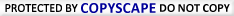### ICFiles SOC 2#### Secure File Transfer Soc 2 starts at \$1 per month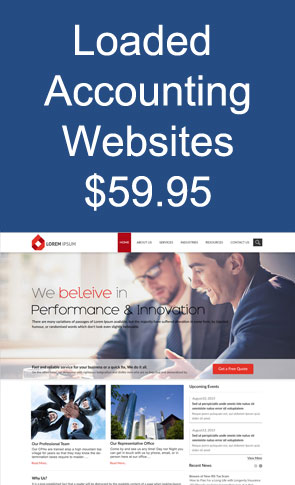#### MyWeb, WordPress1 Click HostingInstall free themes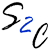Hosting Bundles

Electronic Commerce